Download the eSaral app and start learning from Kota's top IITians and doctors.

# NCERT Solutions for Class 9 Maths chapter 1 Number Systems PDF - eSaral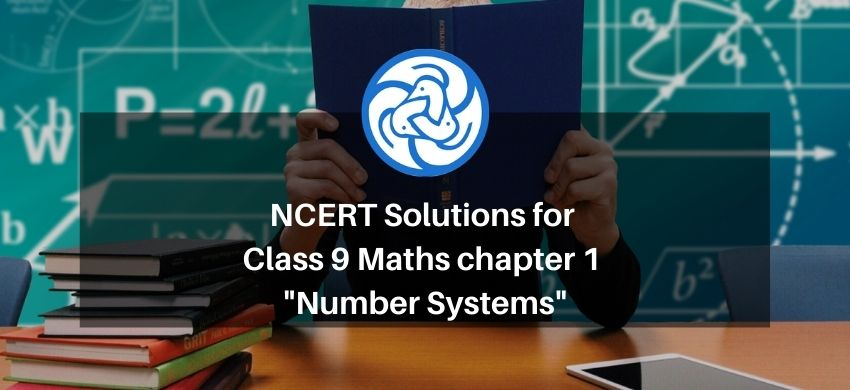# NCERT Solutions for Class 9 Maths chapter 1 Number Systems PDF

Hey, are you a class 9 student and looking for ways to download NCERT Solutions for Class 9 Maths chapter 1 Number Systems pdf ? If yes. Then read this post till the end.

In this article, we have listed NCERT solutions for class 9 Math Chapter 1 Number Systems in PDF that is prepared by Kota’s top IITian’s Faculties by keeping Simplicity in mind.

If you want to learn and understand class 9 Maths chapter 1 "Number Systems" in an easy way then you can use these solutions PDF.

NCERT Solutions helps students to Practice important concepts of subjects easily. Class 9 Maths solutions provide detailed explanations of all the NCERT exercise questions that students can use to clear their doubts instantly.

If you want to score high in your class 9 Maths Exam then it is very important for you to have a good knowledge of all the important topics, so to learn and practice those topics you can use eSaral NCERT Solutions.

So, without wasting more time Let’s start.

### Download NCERT Solutions for Class 9 Maths Chapter 1 Number Systems PDF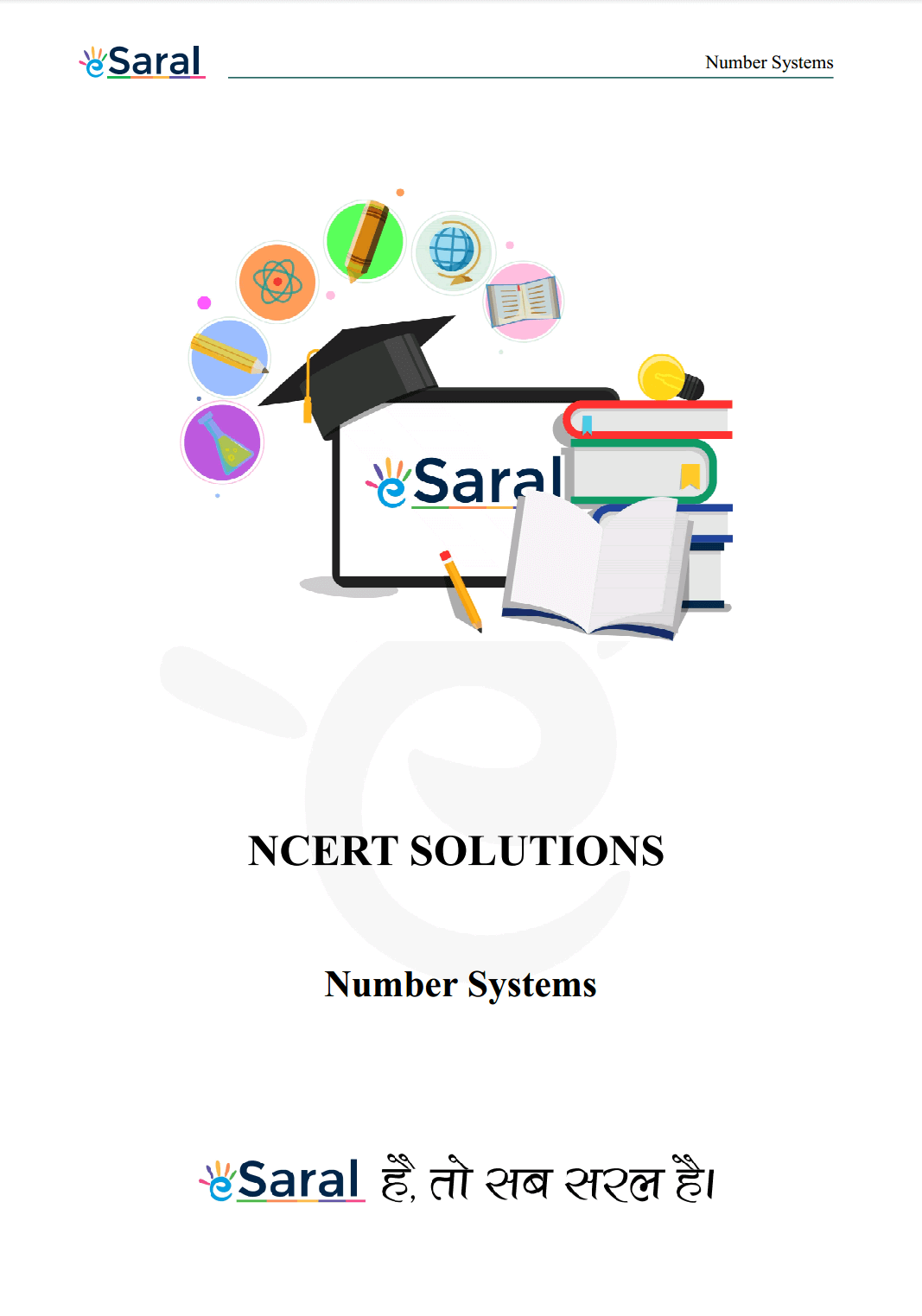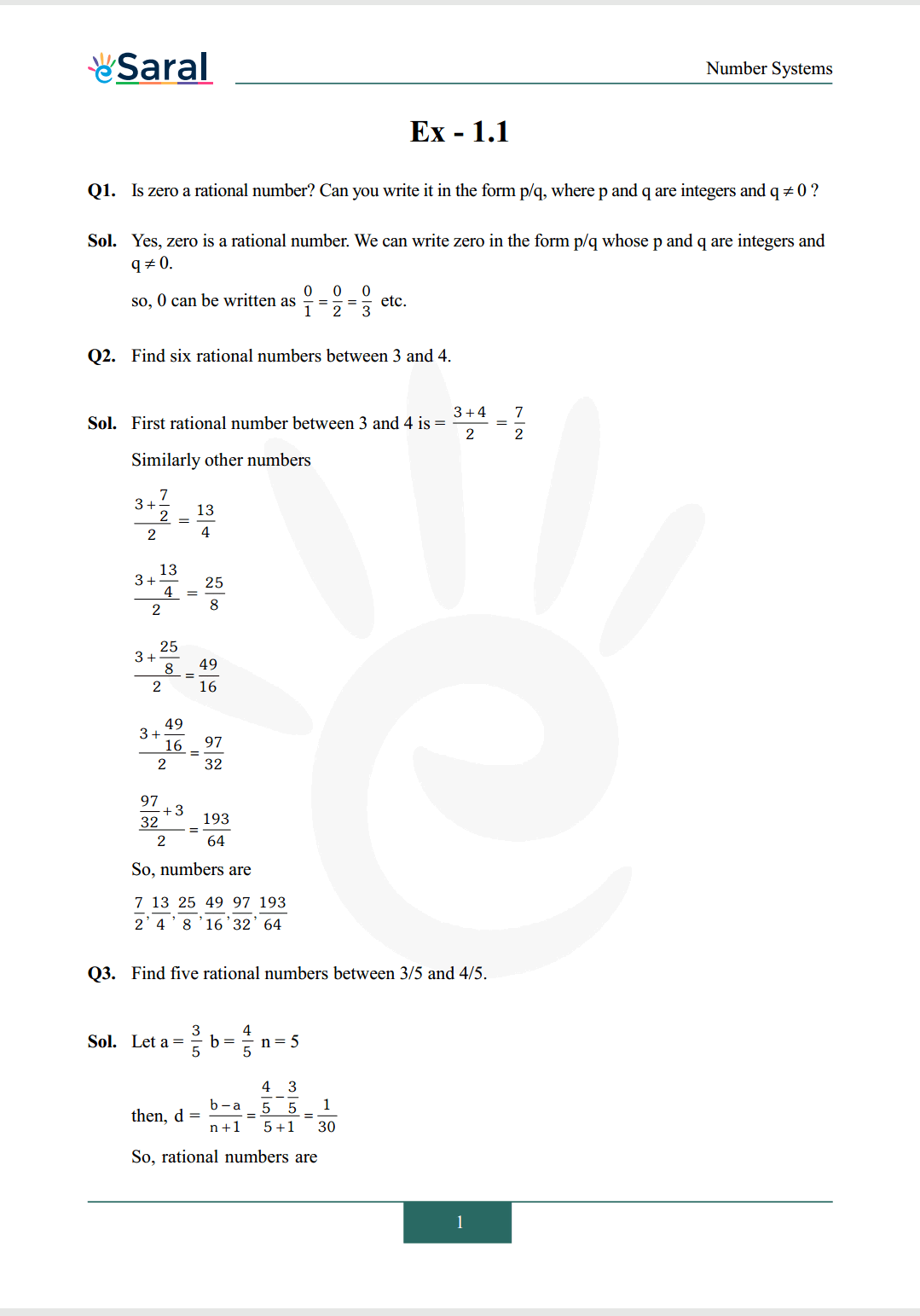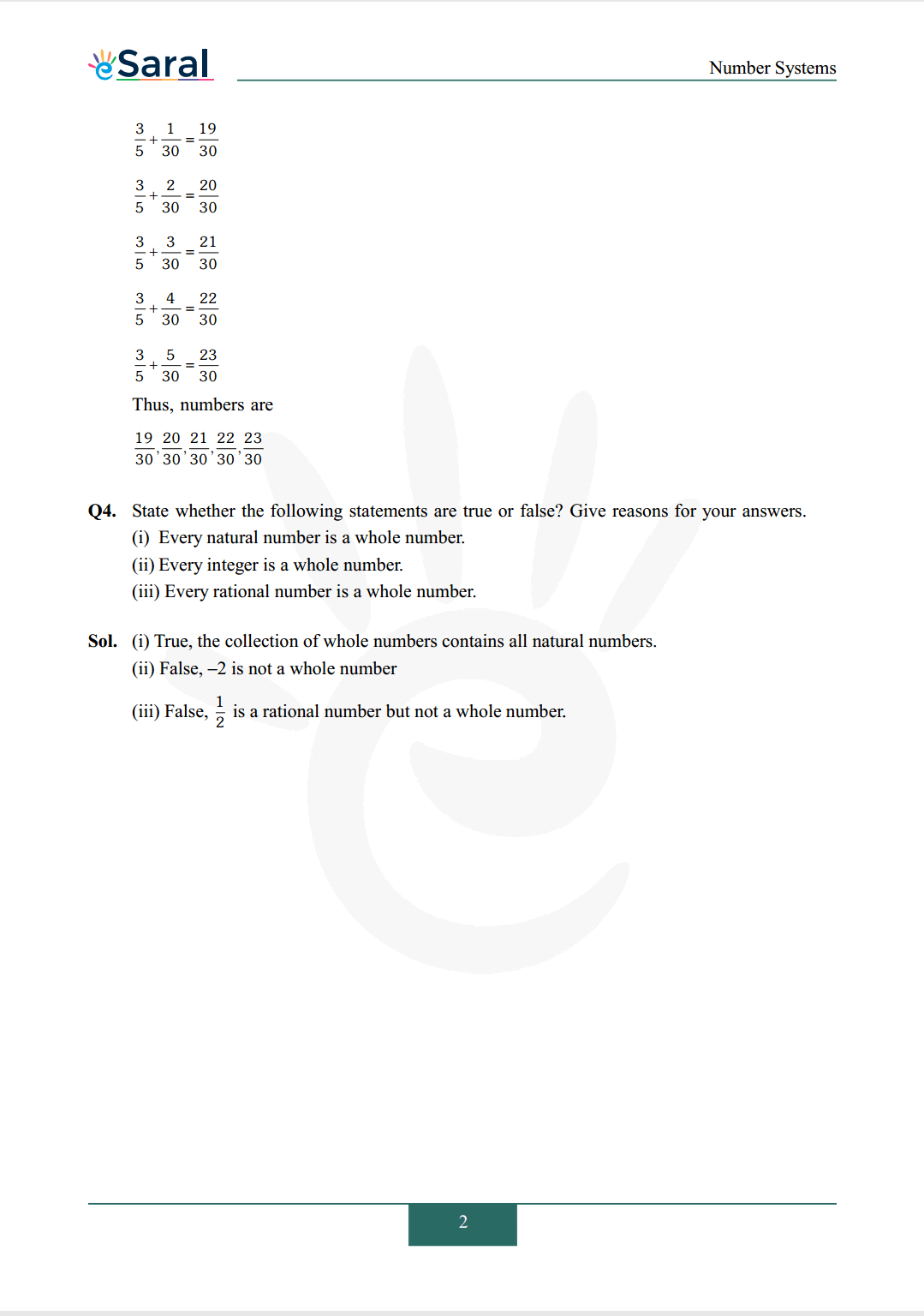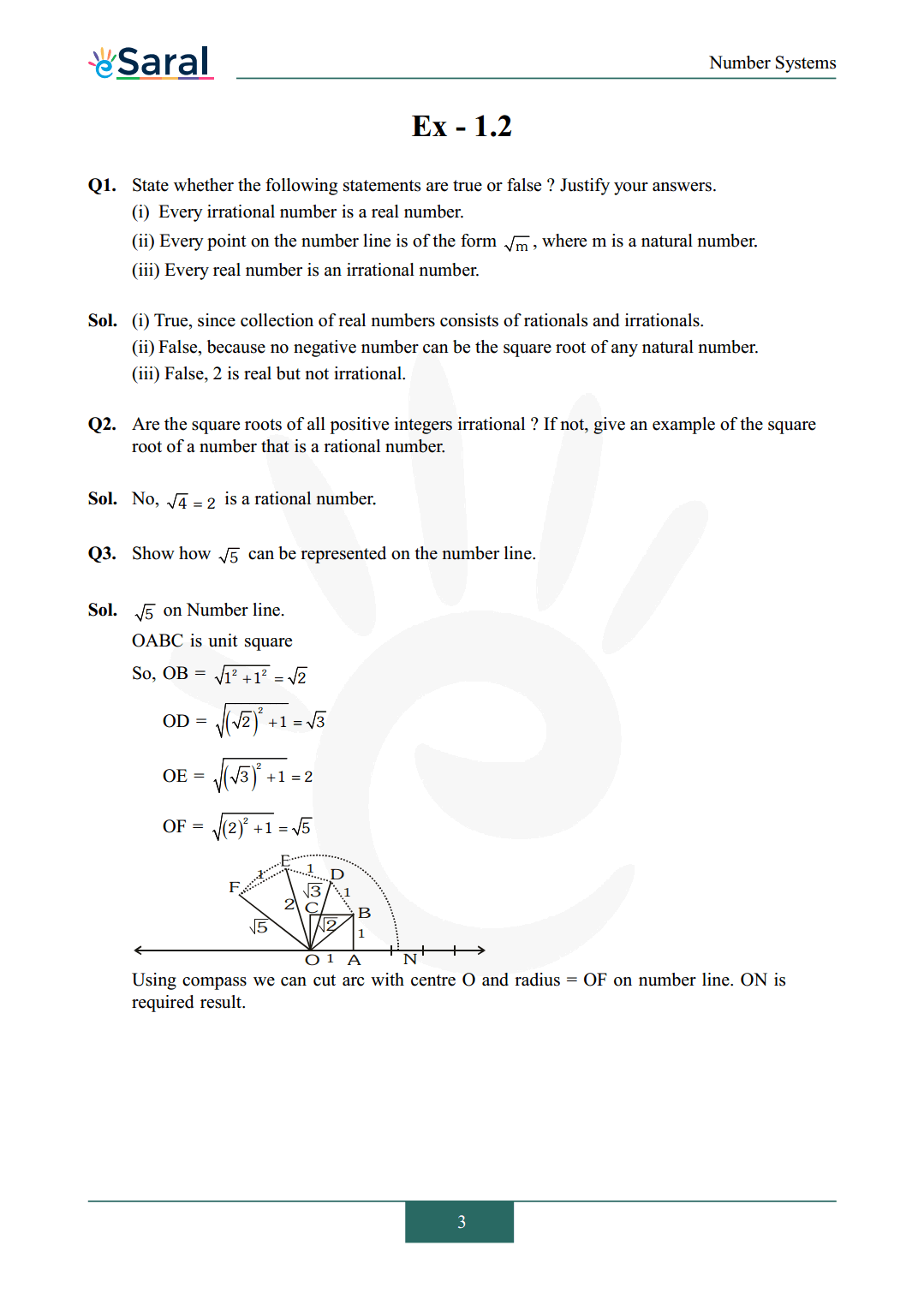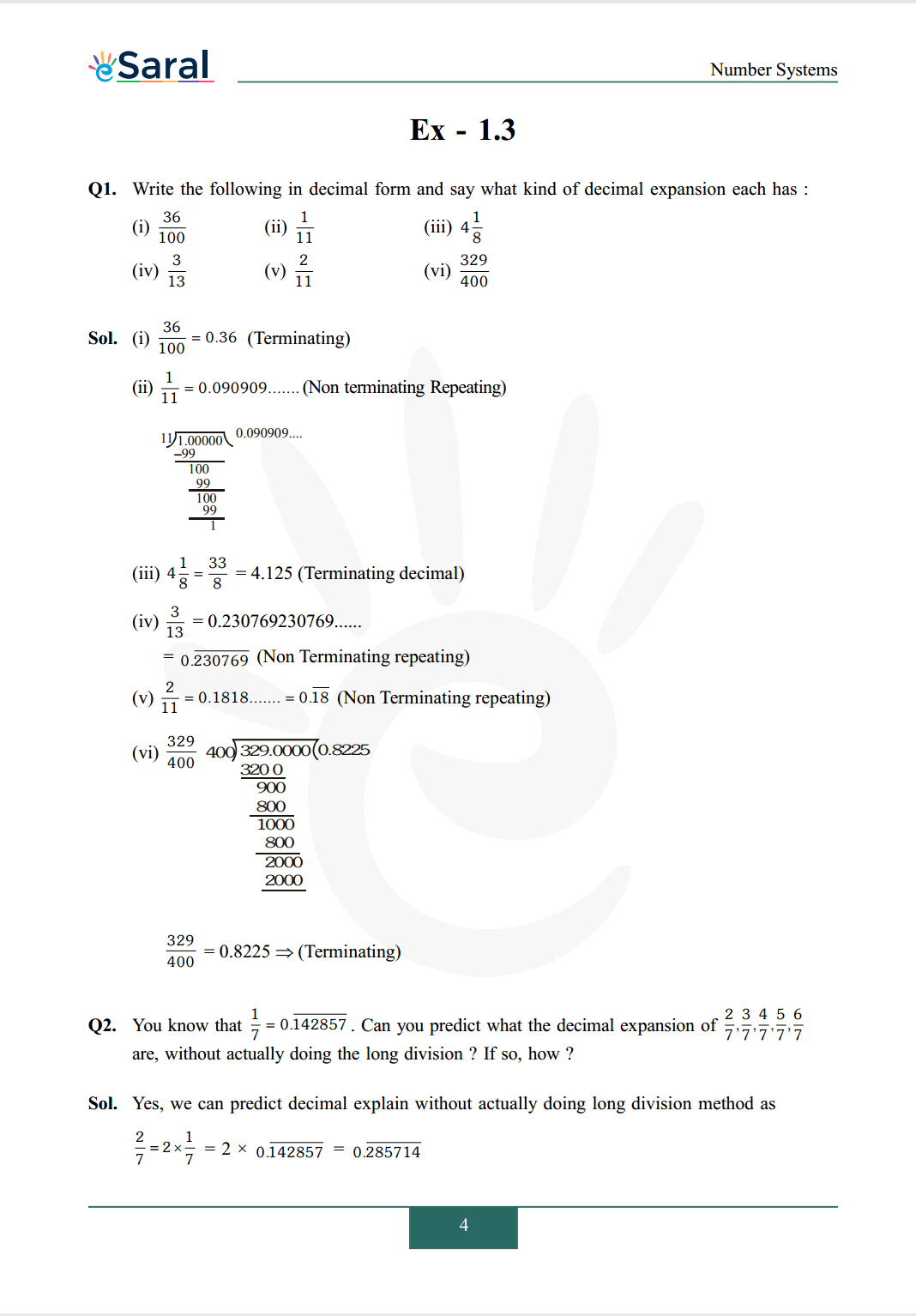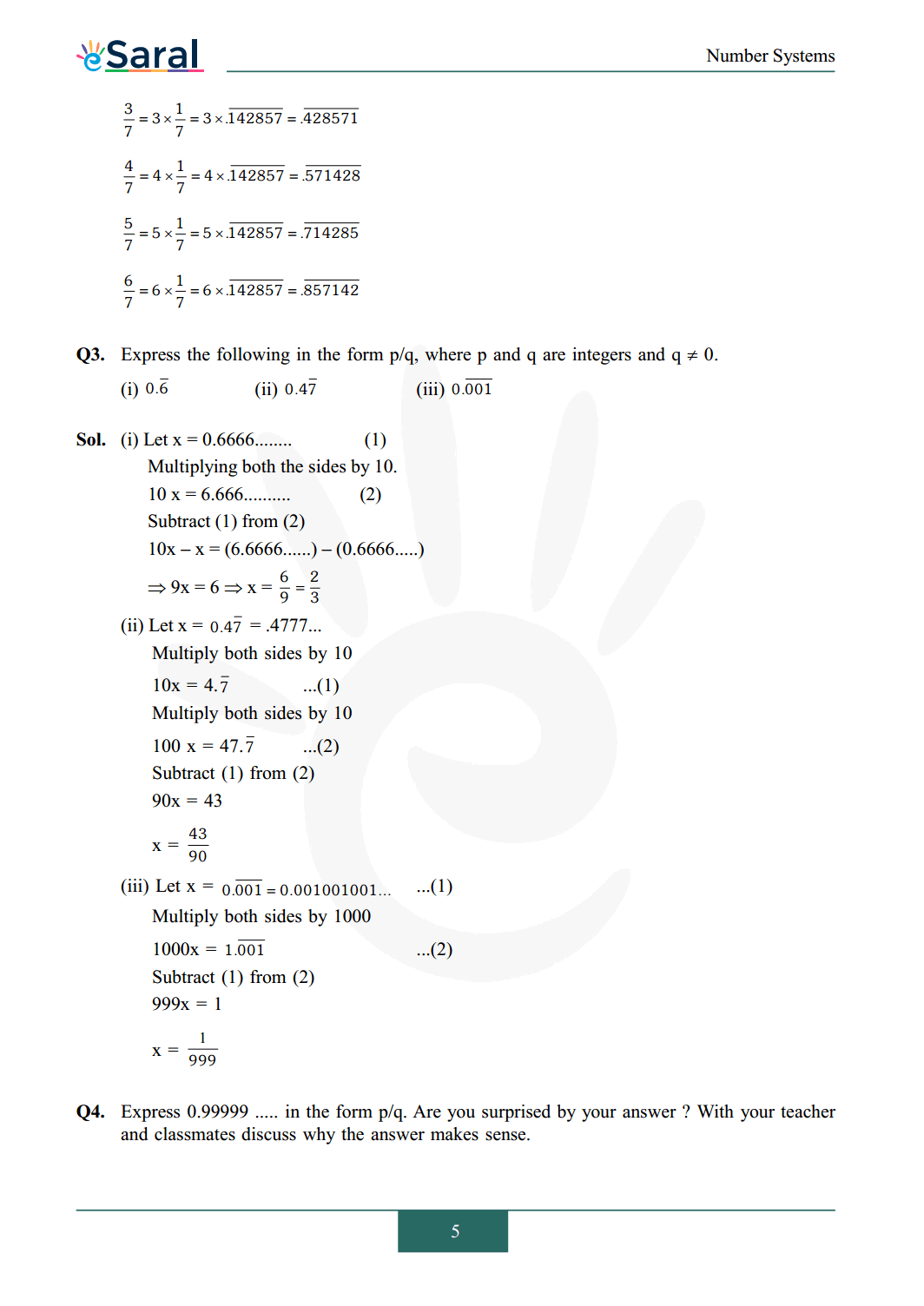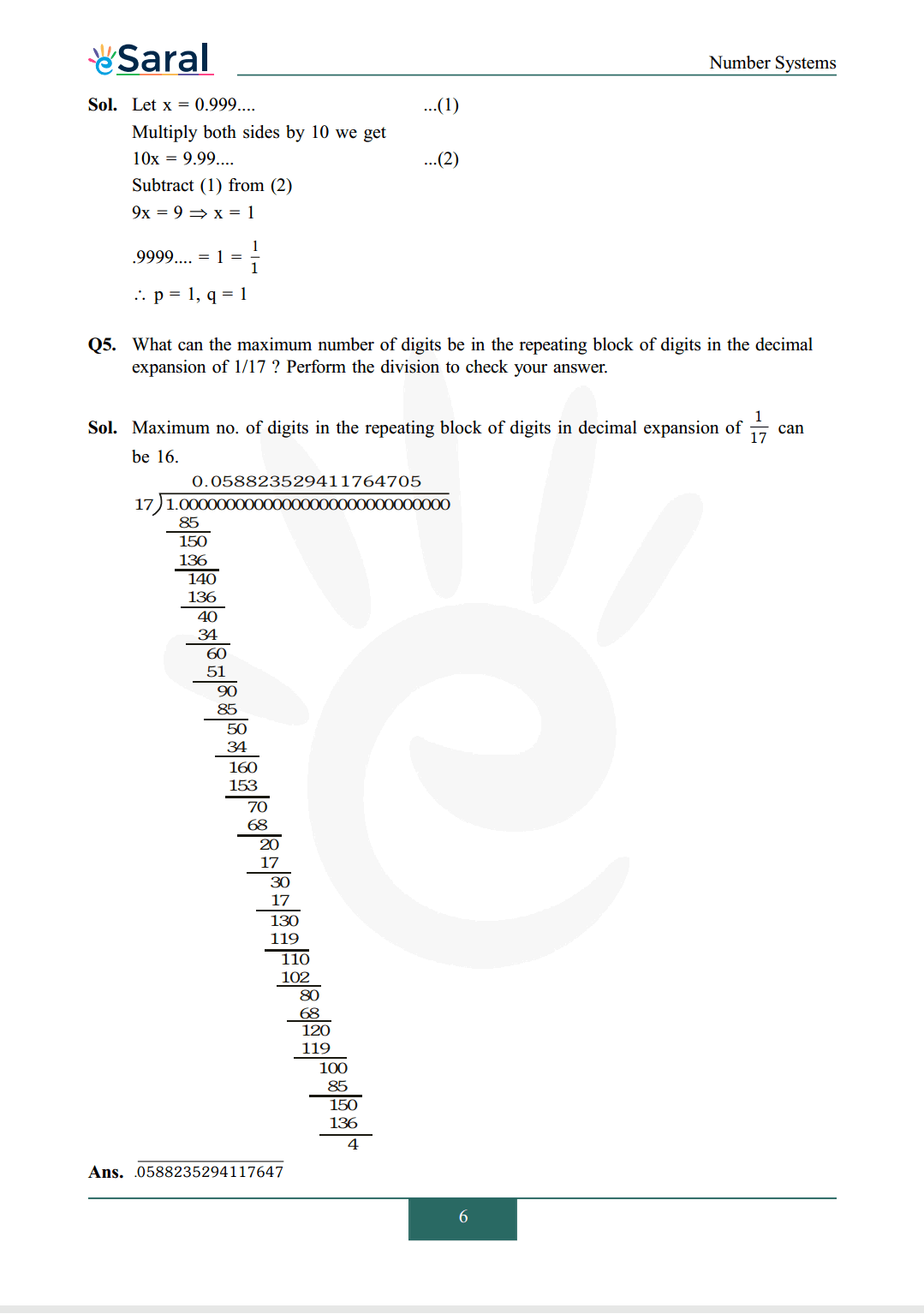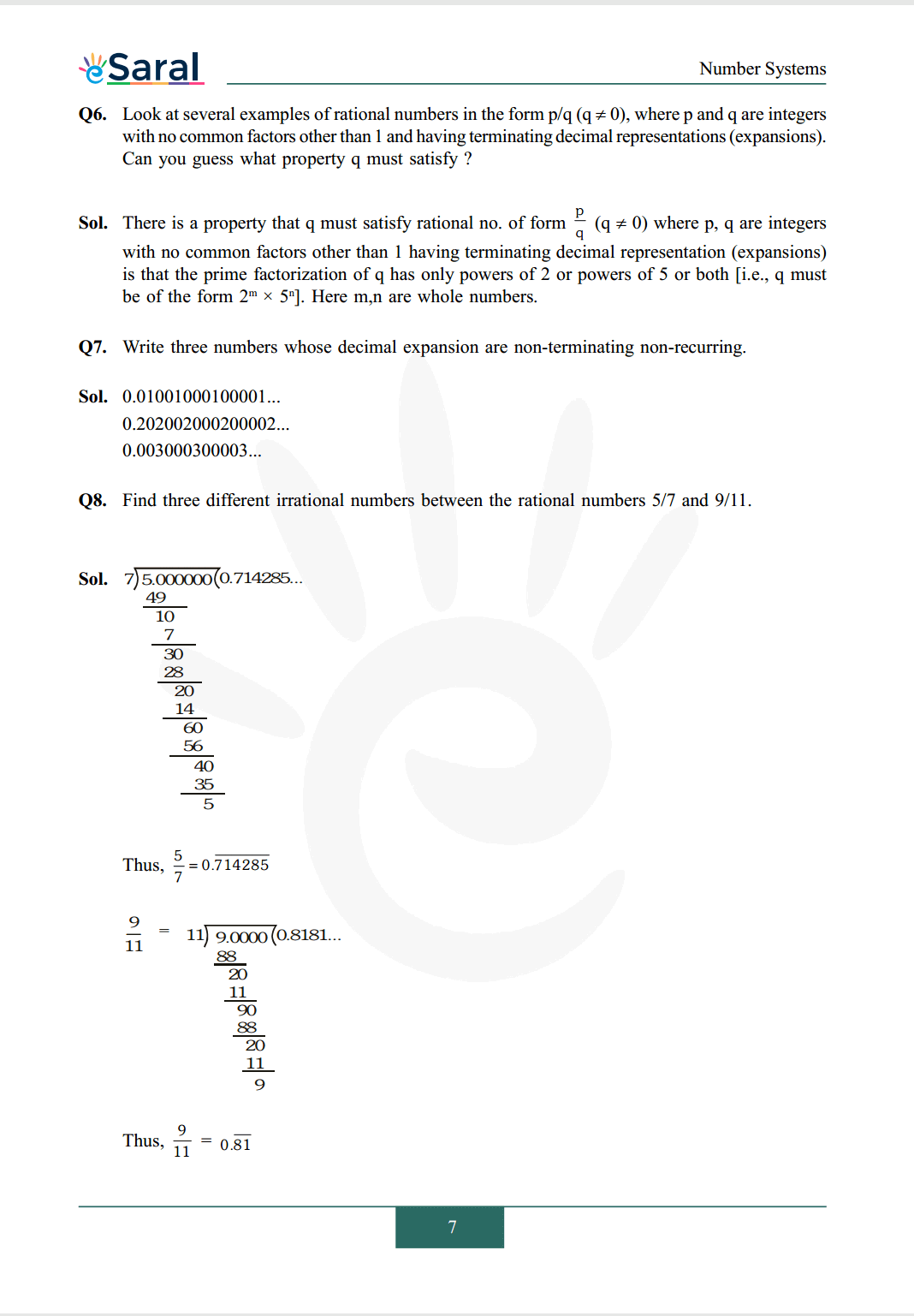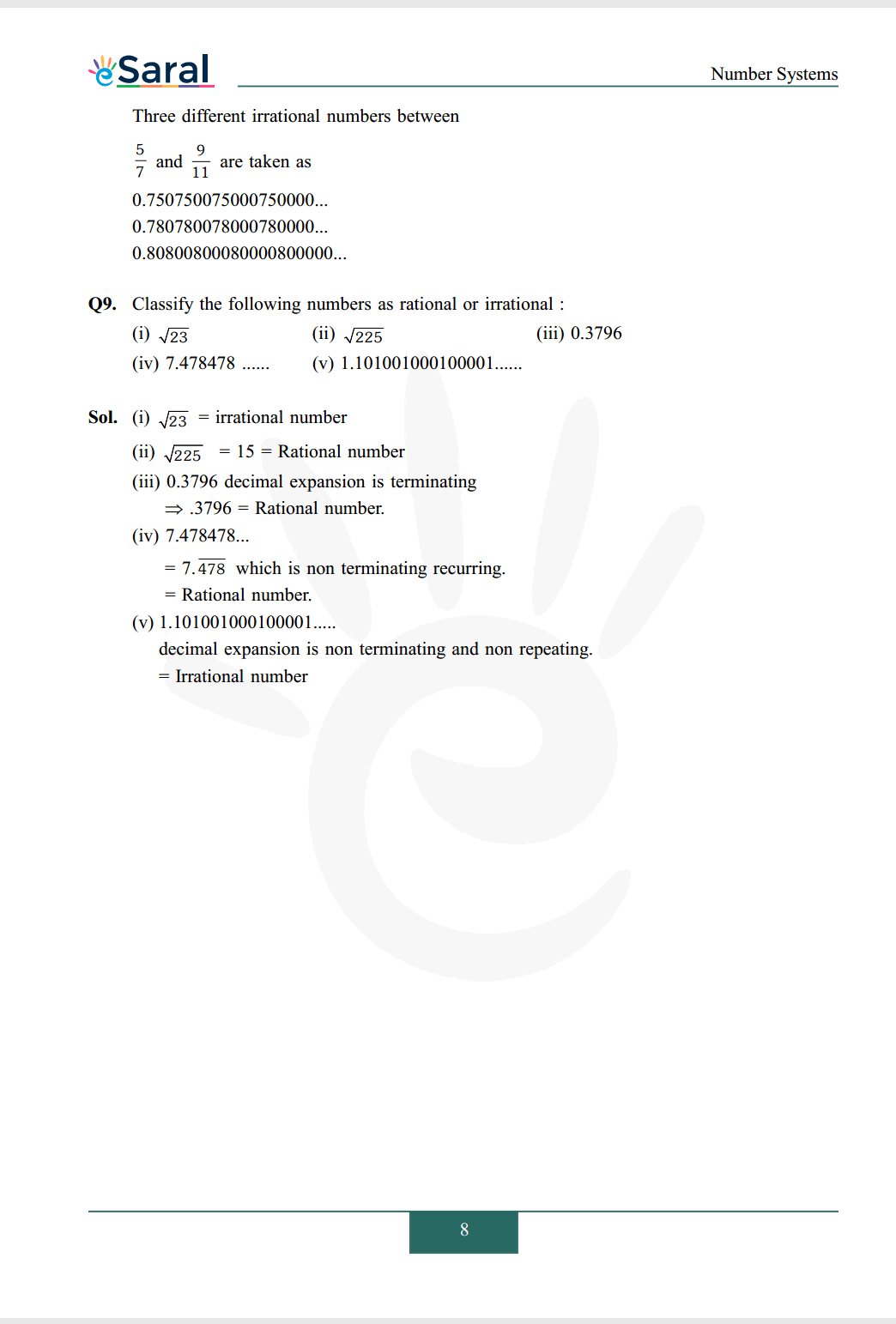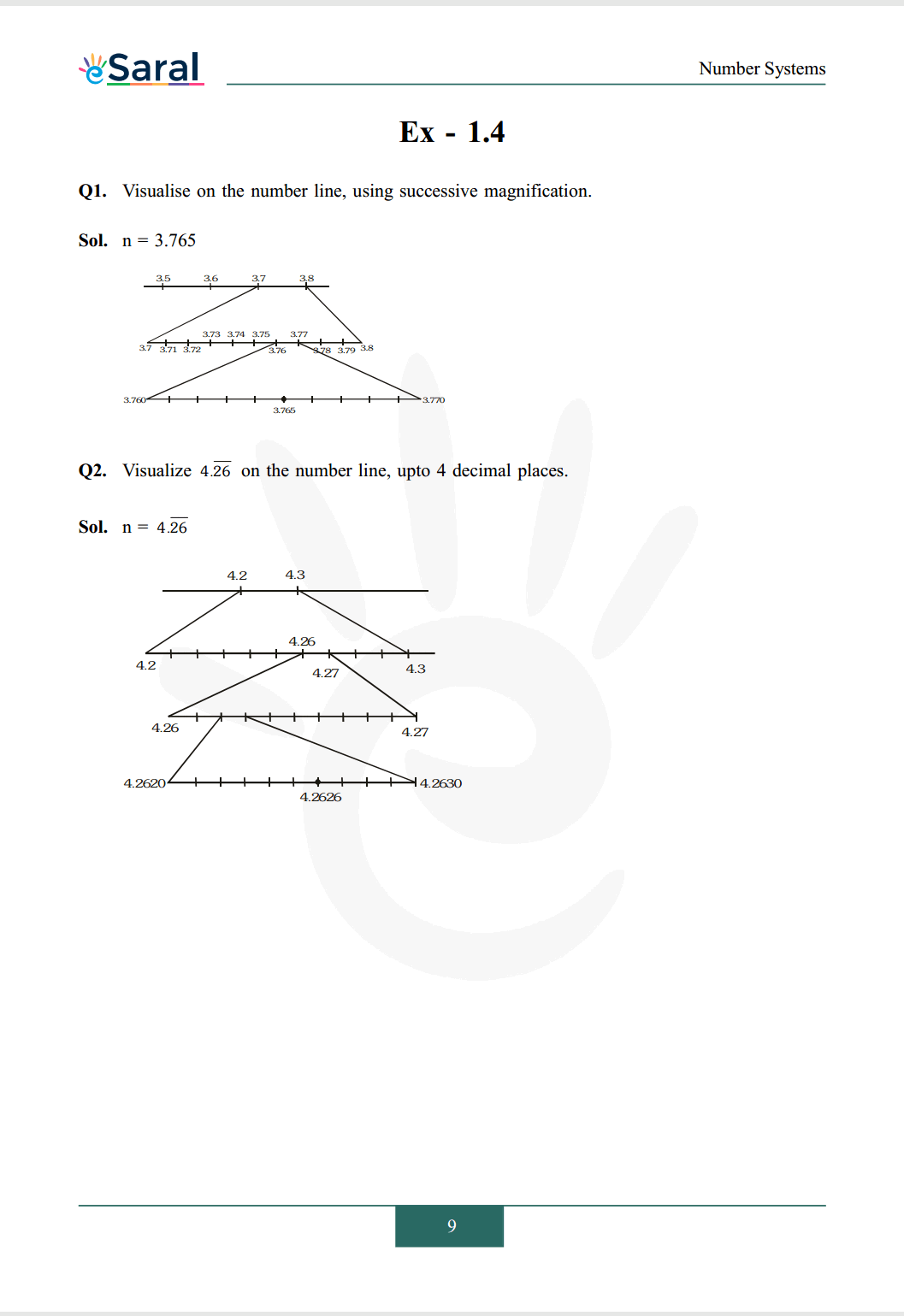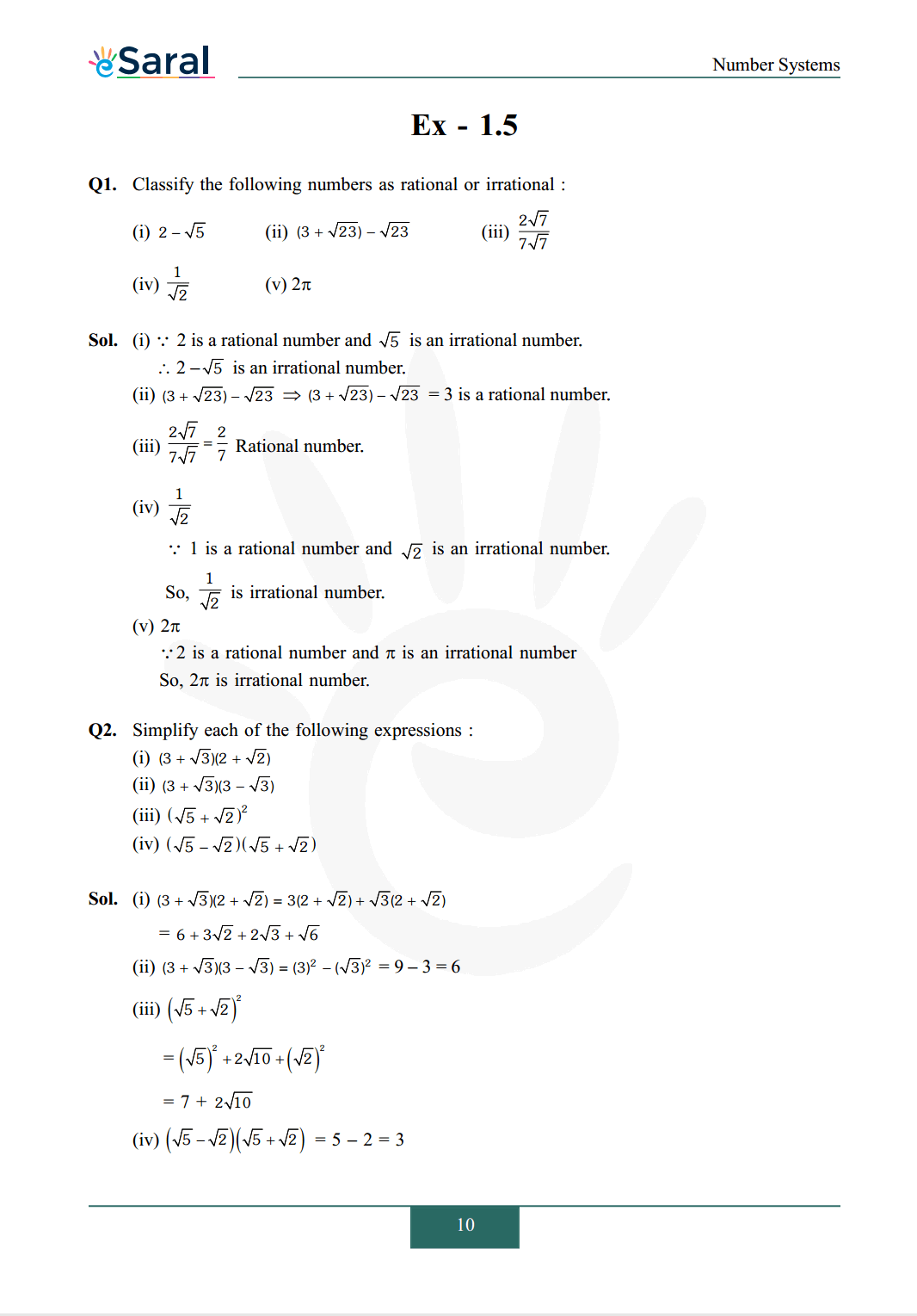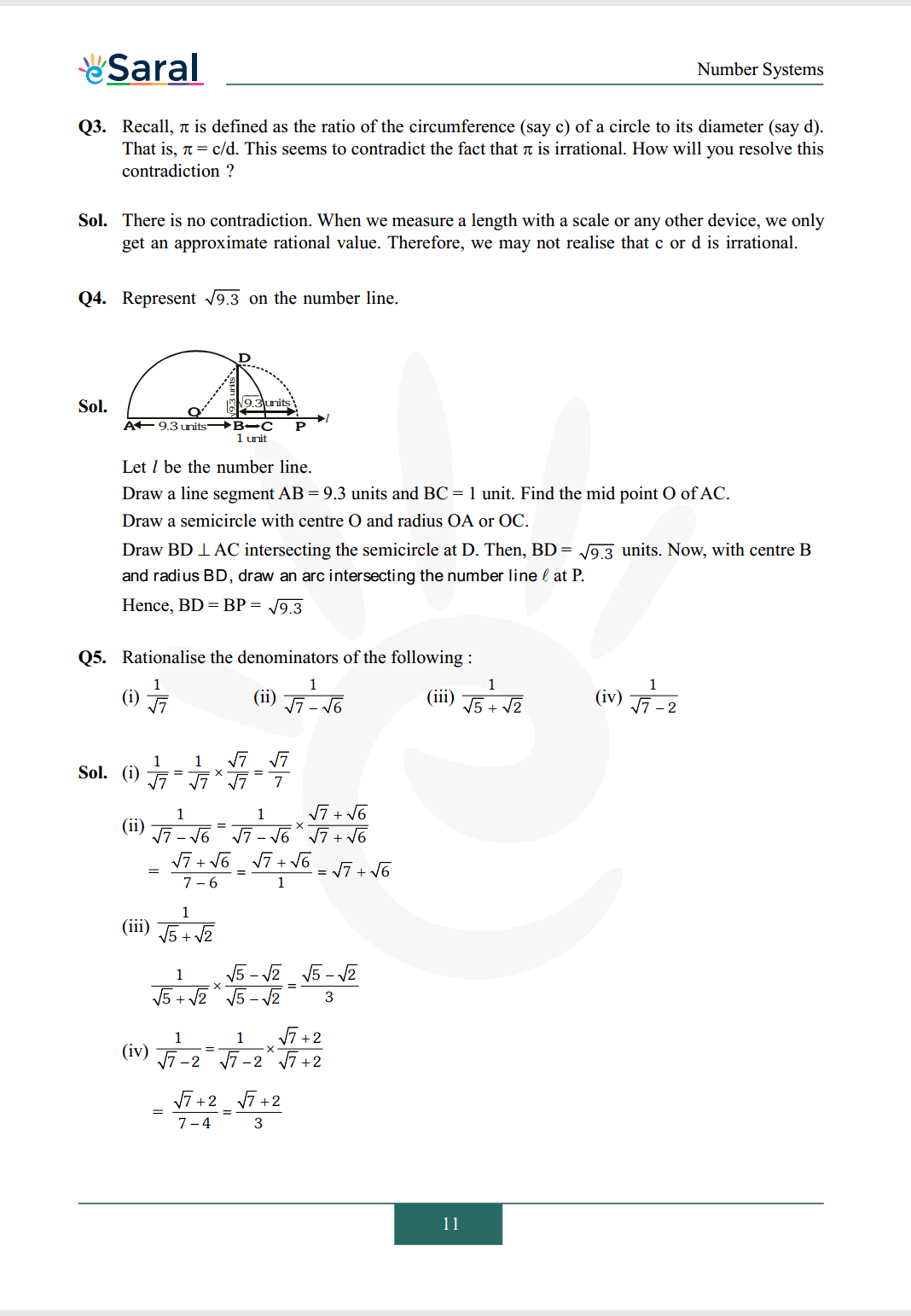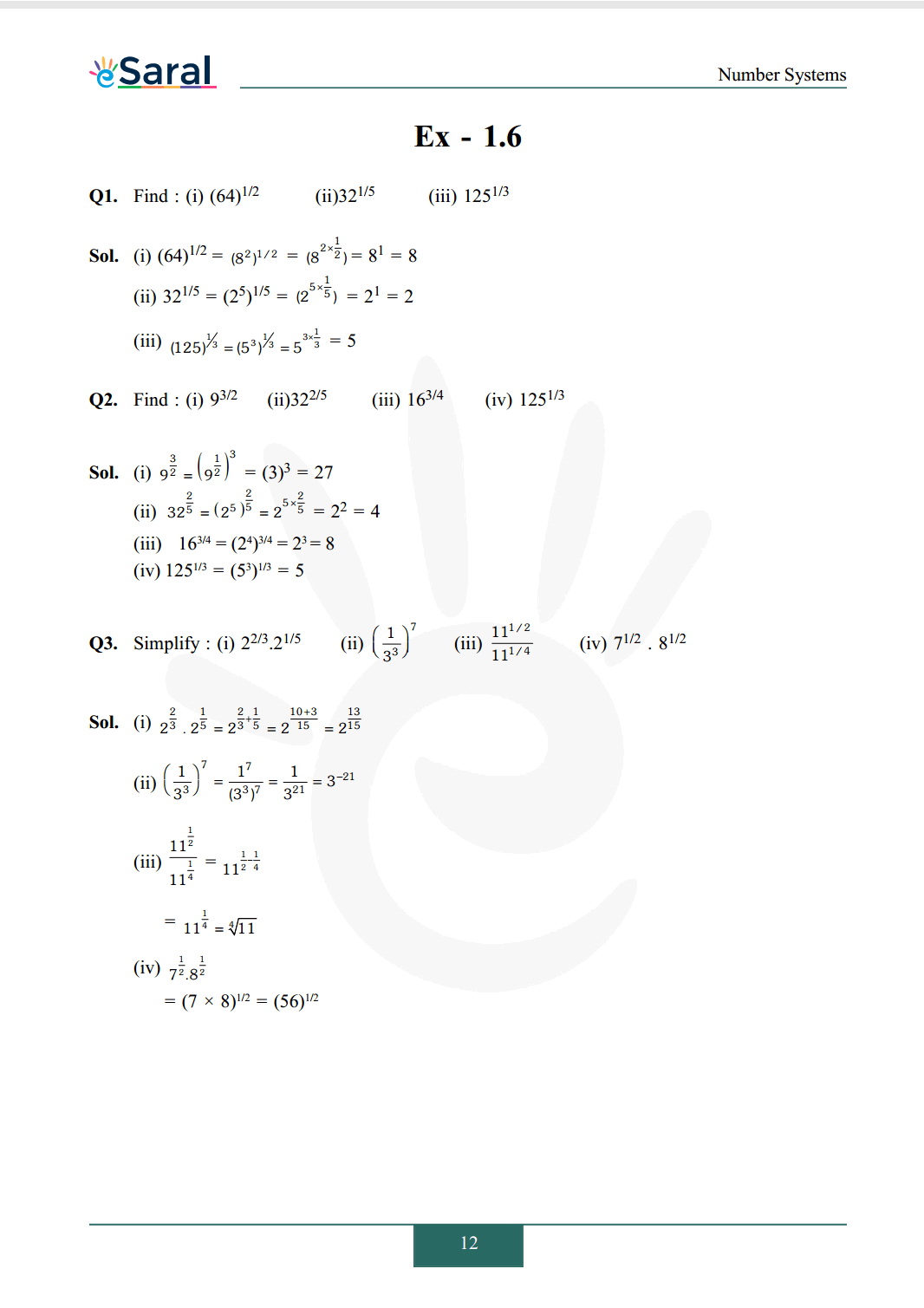### NCERT Class 9 Maths solutions chapter 1 Ex - 1.1

Q1. Is zero a rational number? Can you write it in the form $\mathrm{p} / \mathrm{q}$, where $\mathrm{p}$ and $\mathrm{q}$ are integers and $\mathrm{q} \neq 0$ ?

Sol. Yes, zero is a rational number. We can write zero in the form $\mathrm{p} / \mathrm{q}$ whose $\mathrm{p}$ and $\mathrm{q}$ are integers and $\mathrm{q} \neq 0$
so, 0 can be written as $\frac{0}{1}=\frac{0}{2}=\frac{0}{3}$ etc.

Q2. Find six rational numbers between 3 and 4.

Sol. First rational number between 3 and 4 is $=\frac{3+4}{2}=\frac{7}{2}$
Similarly other numbers
$\frac{3+\frac{7}{2}}{2}=\frac{13}{4}$
$\frac{3+\frac{13}{4}}{2}=\frac{25}{8}$
$\frac{3+\frac{25}{8}}{2}=\frac{49}{16}$
$\frac{3+\frac{49}{16}}{2}=\frac{97}{32}$
$\frac{\frac{97}{32}+3}{2}=\frac{193}{64}$
So, numbers are
$\frac{7}{2}, \frac{13}{4}, \frac{25}{8}, \frac{49}{16}, \frac{97}{32}, \frac{193}{64}$

Q3. Find five rational numbers between $3 / 5$ and $4 / 5$.

Sol. Let $\mathrm{a}=\frac{3}{5} \mathrm{~b}=\frac{4}{5} \mathrm{n}=5$
then, $\mathrm{d}=\frac{\mathrm{b}-\mathrm{a}}{\mathrm{n}+1}=\frac{\frac{4}{5}-\frac{3}{5}}{5+1}=\frac{1}{30}$
So, rational numbers are
$\frac{3}{5}+\frac{1}{30}=\frac{19}{30}$
$\frac{3}{5}+\frac{2}{30}=\frac{20}{30}$
$\frac{3}{5}+\frac{3}{30}=\frac{21}{30}$
$\frac{3}{5}+\frac{4}{30}=\frac{22}{30}$
$\frac{3}{5}+\frac{5}{30}=\frac{23}{30}$
Thus, numbers are
$\frac{19}{30}, \frac{20}{30}, \frac{21}{30}, \frac{22}{30}, \frac{23}{30}$

Q4. State whether the following statements are true or false? Give reasons for your answers.
(i) Every natural number is a whole number.
(ii) Every integer is a whole number.
(iii) Every rational number is a whole number.

Sol. (i) True, the collection of whole numbers contains all natural numbers.
(ii) False, $-2$ is not a whole number
(iii) False, $\frac{1}{2}$ is a rational number but not a whole number.

### NCERT Class 9 Maths solutions chapter 1 Ex - 1.2

Q1. State whether the following statements are true or false ? Justify your answers.

(i) Every irrational number is a real number.
(ii) Every point on the number line is of the form $\sqrt{m}$, where $m$ is a natural number.
(iii) Every real number is an irrational number.

Sol. (i) True, since collection of real numbers consists of rationals and irrationals.
(ii) False, because no negative number can be the square root of any natural number.
(iii) False, 2 is real but not irrational.

Q2. Are the square roots of all positive integers irrational ? If not, give an example of the square root of a number that is a rational number.

Sol. No, $\sqrt{4}=2$ is a rational number.

Q3. Show how $\sqrt{5}$ can be represented on the number line.

Sol. $\sqrt{5}$ on Number line.
$\mathrm{OABC}$ is unit square
So, $\mathrm{OB}=\sqrt{1^{2}+1^{2}}=\sqrt{2}$
$\mathrm{OD}=\sqrt{(\sqrt{2})^{2}+1}=\sqrt{3}$
$\mathrm{OE}=\sqrt{(\sqrt{3})^{2}+1}=2$
$\mathrm{OF}=\sqrt{(2)^{2}+1}=\sqrt{5}$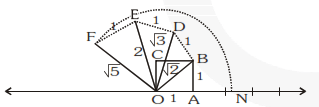Using compass we can cut arc with centre $\mathrm{O}$ and radius $=\mathrm{OF}$ on number line. $\mathrm{ON}$ is required result.

### NCERT Class 9 Maths solutions chapter 1 Ex - 1.3

Q1. Write the following in decimal form and say what kind of decimal expansion each has:

(i) $\frac{36}{100}$
(ii) $\frac{1}{11}$
(iii) $4 \frac{1}{8}$
(iv) $\frac{3}{13}$
(v) $\frac{2}{11}$
(vi) $\frac{329}{400}$

Sol. (i) $\frac{36}{100}=0.36$ (Terminating)
(ii) $\frac{1}{11}=0.090909 \ldots \ldots$ (Non terminating Repeating)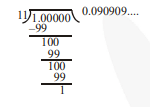(iii) $4 \frac{1}{8}=\frac{33}{8}=4.125$ (Terminating decimal)
(iv) $\frac{3}{13}=0.230769230769 \ldots \ldots$
$=0 . \overline{230769}$ (Non Terminating repeating)
(v) $\frac{2}{11}=0.1818 \ldots \ldots .0 . \overline{8}$ (Non Terminating repeating)
(vi) $\frac{329}{400}$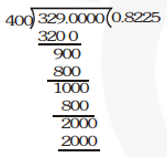$\frac{329}{400}=0.8225 \Rightarrow$ (Terminating)

Q2. You know that $\frac{1}{7}=0 . \overline{142857}$. Can you predict what the decimal expansion of $\frac{2}{7}, \frac{3}{7}, \frac{4}{7}, \frac{5}{7}, \frac{6}{7}$

Sol. Yes, we can predict decimal explain without actually doing long division method as
$\frac{2}{7}=2 \times \frac{1}{7}$
$=2 \times 0 . \overline{142857}=0 . \overline{285714}$
$\frac{3}{7}=3 \times \frac{1}{7}$
$=3 \times \overline{142857}=\overline{428571}$
$\frac{4}{7}=4 \times \frac{1}{7}$
$=4 \times \overline{142857}=\overline{571428}$
$\frac{5}{7}=5 \times \frac{1}{7}$
$=5 \times \overline{142857}=\overline{.714285}$
$\frac{6}{7}=6 \times \frac{1}{7}$
$=6 \times \overline{142857}=\overline{.857142}$

Q3. Express the following in the form $\mathrm{p} / \mathrm{q}$, where $\mathrm{p}$ and $\mathrm{q}$ are integers and $\mathrm{q} \neq 0$.
(i) $0 . \overline{6}$
(ii) $0.4 \overline{7}$
(iii) $0 . \overline{001}$

Sol. (i) Let $\mathrm{x}=0.6666 \ldots \ldots \ldots$(1)
Multiplying both the sides by 10 .
$10 \mathrm{x}=6.666 \ldots \ldots \ldots$(2)
Subtract (1) from (2)
$10 \mathrm{x}-\mathrm{x}=(6.6666 \ldots \ldots)-(0.6666 \ldots . .)$
$\Rightarrow 9 x=6 \Rightarrow x=\frac{6}{9}=\frac{2}{3}$
(ii) Let $\mathrm{x}=0.4 \overline{7}=.4777 \ldots$
Multiply both sides by 10
$10 \mathrm{x}=4 . \overline{7}$$\ldots(1) Multiply both sides by 10 100 \mathrm{x}=47 . \overline{7}... (2) Subtract (1) from (2) 90 \mathrm{x}=43 x=\frac{43}{90} (iii) Let \mathrm{x}=0 . \overline{001}=0.001001001 \ldots . . .(1) Multiply both sides by 1000 1000 \mathrm{x}=1 . \overline{001}$$\ldots(2)$
Subtract (1) from (2)
$999 x=1$
$\mathrm{x}=\frac{1}{999}$’

Q4. Express $0.99999 \ldots . .$ in the form $\mathrm{p} / \mathrm{q}$. Are you surprised by your answer? With your teacher and classmates discuss why the answer makes sense.

Sol. Let $\mathrm{x}=0.999 \ldots .$(1)
Multiply both sides by 10 we get
$10 \mathrm{x}=9.99 \ldots$...(2)
Subtract (1) from (2)
$9 x=9 \Rightarrow x=1$
$.9999 \ldots .=1=\frac{1}{1}$
$\therefore \mathrm{p}=1, \mathrm{q}=1$

Q5. What can the maximum number of digits be in the repeating block of digits in the decimal expansion of $1 / 17$ ? Perform the division to check your answer.

Sol. Maximum no. of digits in the repeating block of digits in decimal expansion of $\frac{1}{17}$ can be 16 .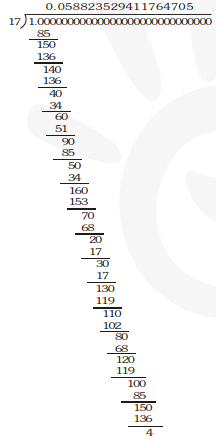Ans. $\overline{0588235294117647}$

Q6. Look at several examples of rational numbers in the form $\mathrm{p} / \mathrm{q}(\mathrm{q} \neq 0)$, where $\mathrm{p}$ and $\mathrm{q}$ are integers with no common factors other than 1 and having terminating decimal representations (expansions). Can you guess what property q must satisfy?

Sol. There is a property that q must satisfy rational no. of form $\frac{p}{q}(q \neq 0)$ where $p, q$ are integerswith no common factors other than 1 having terminating decimal representation (expansions) is that the prime factorization of $q$ has only powers of 2 or powers of 5 or both [i.e., $q$ must be of the form $\left.2^{\mathrm{m}} \times 5^{\mathrm{n}}\right]$. Here $\mathrm{m}, \mathrm{n}$ are whole numbers.

Q7. Write three numbers whose decimal expansion are non-terminating non-recurring.

Sol. $0.01001000100001 \ldots$
$0.202002000200002 \ldots$
$0.003000300003 \ldots$

Q8. Find three different irrational numbers between the rational numbers $5 / 7$ and $9 / 11$.
Sol.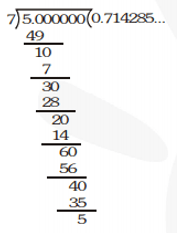Thus, $\frac{5}{7}=0 . \overline{714285}$
$\frac{9}{11}=$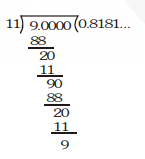Thus, $\frac{9}{11}=0 . \overline{81}$
Three different irrational numbers between
$\frac{5}{7}$ and $\frac{9}{11}$ are taken as
$0.750750075000750000 \ldots$
$0.780780078000780000 \ldots$
$0.80800800080000800000 \ldots$

Q9. Classify the following numbers as rational or irrational:
(i) $\sqrt{23}$
(ii) $\sqrt{225}$
(iii) $0.3796$
(iv) $7.478478 \ldots \ldots$
(v) $1.101001000100001 \ldots \ldots$

Sol. (i) $\sqrt{23}=$ irrational number
(ii) $\sqrt{225}=15=$ Rational number
(iii) $0.3796$ decimal expansion is terminating
$\Rightarrow .3796=$ Rational number.
(iv) $7.478478 \ldots$
$=7 . \overline{478}$ which is non terminating recurring.
$=$ Rational number.
(v) $1.101001000100001 \ldots .$
decimal expansion is non terminating and non repeating.
$=$ Irrational number

### NCERT Class 9 Maths solutions chapter 1 Ex - 1.4

Q1. Visualise on the number line, using successive magnification.

Sol. $\mathrm{n}=3.765$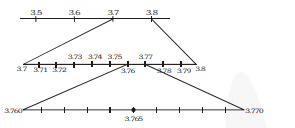Q2. Visualize $4 . \overline{26}$ on the number line, upto 4 decimal places.

Sol. $\mathrm{n}=4 \overline{26}$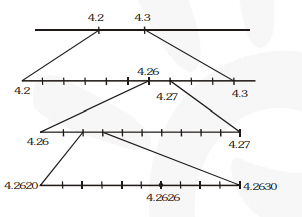### NCERT Class 9 Maths solutions chapter 1 Ex - 1.5

Q1. Classify the following numbers as rational or irrational:

(i) $2-\sqrt{5}$
(ii) $(3+\sqrt{23})-\sqrt{23}$
(iii) $\frac{2 \sqrt{7}}{7 \sqrt{7}}$
(iv) $\frac{1}{\sqrt{2}}$
(v) $2 \pi$

Sol. (i) $\because 2$ is a rational number and $\sqrt{5}$ is an irrational number.
$\therefore 2-\sqrt{5}$ is an irrational number.
(ii) $(3+\sqrt{23})-\sqrt{23} \Rightarrow(3+\sqrt{23})-\sqrt{23}$
$=3$ is a rational number.
(iii) $\frac{2 \sqrt{7}}{7 \sqrt{7}}=\frac{2}{7}$ Rational number.
(iv) $\frac{1}{\sqrt{2}}$
$\because 1$ is a rational number and $\sqrt{2}$ is an irrational number.
So, $\frac{1}{\sqrt{2}}$ is irrational number.
(v) $2 \pi$
$\because 2$ is a rational number and $\pi$ is an irrational number
So, $2 \pi$ is irrational number.
Q2. Simplify each of the following expressions:
(i) $(3+\sqrt{3})(2+\sqrt{2})$
(ii) $(3+\sqrt{3})(3-\sqrt{3})$
(iii) $(\sqrt{5}+\sqrt{2})^{2}$
(iv) $(\sqrt{5}-\sqrt{2})(\sqrt{5}+\sqrt{2})$

Sol. (i) $(3+\sqrt{3})(2+\sqrt{2})=3(2+\sqrt{2})+\sqrt{3}(2+\sqrt{2})$
$=6+3 \sqrt{2}+2 \sqrt{3}+\sqrt{6}$
(ii) $(3+\sqrt{3})(3-\sqrt{3})=(3)^{2}-(\sqrt{3})^{2}=9-3=6$
(iii) $(\sqrt{5}+\sqrt{2})^{2}$
$=(\sqrt{5})^{2}+2 \sqrt{10}+(\sqrt{2})^{2}$
$=7+2 \sqrt{10}$
(iv) $(\sqrt{5}-\sqrt{2})(\sqrt{5}+\sqrt{2})=5-2=3$

Q3. Recall, $\pi$ is defined as the ratio of the circumference (say c) of a circle to its diameter (say d). That is, $\pi=\mathrm{c} / \mathrm{d}$. This seems to contradict the fact that $\pi$ is irrational. How will you resolve this contradiction?

Sol. There is no contradiction. When we measure a length with a scale or any other device, we only get an approximate rational value. Therefore, we may not realise that $\mathrm{c}$ or $\mathrm{d}$ is irrational.

Q4. Represent $\sqrt{9.3}$ on the number line.

Sol.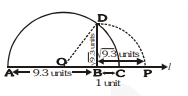Let $l$ be the number line.
Draw a line segment $\mathrm{AB}=9.3$ units and $\mathrm{BC}=1$ unit. Find the mid point $\mathrm{O}$ of $\mathrm{AC}$.
Draw a semicircle with centre $\mathrm{O}$ and radius $\mathrm{OA}$ or $\mathrm{OC}$.
Draw BD $\perp$ AC intersecting the semicircle at $D$. Then, $B D=\sqrt{9.3}$ units. Now, with centre $B$
and radius BD, draw an arc intersecting the number line $\ell$ at $\mathrm{P}$.
Hence, $\mathrm{BD}=\mathrm{BP}=\sqrt{9.3}$

Q5. Rationalise the denominators of the following:
(i) $\frac{1}{\sqrt{7}}$
(ii) $\frac{1}{\sqrt{7}-\sqrt{6}}$
(iii) $\frac{1}{\sqrt{5}+\sqrt{2}}$
(iv) $\frac{1}{\sqrt{7}-2}$

Sol.(i) $\frac{1}{\sqrt{7}}=\frac{1}{\sqrt{7}} \times \frac{\sqrt{7}}{\sqrt{7}}=\frac{\sqrt{7}}{7}$
(ii) $\frac{1}{\sqrt{7}-\sqrt{6}}=\frac{1}{\sqrt{7}-\sqrt{6}} \times \frac{\sqrt{7}+\sqrt{6}}{\sqrt{7}+\sqrt{6}}$
$=\frac{\sqrt{7}+\sqrt{6}}{7-6}=\frac{\sqrt{7}+\sqrt{6}}{1}=\sqrt{7}+\sqrt{6}$
(iii) $\frac{1}{\sqrt{5}+\sqrt{2}}$
$\frac{1}{\sqrt{5}+\sqrt{2}} \times \frac{\sqrt{5}-\sqrt{2}}{\sqrt{5}-\sqrt{2}}=\frac{\sqrt{5}-\sqrt{2}}{3}$
(iv) $\frac{1}{\sqrt{7}-2}=\frac{1}{\sqrt{7}-2} \times \frac{\sqrt{7}+2}{\sqrt{7}+2}$
$=\frac{\sqrt{7}+2}{7-4}=\frac{\sqrt{7}+2}{3}$

### NCERT Class 9 Maths solutions chapter 1 Ex - 1.6

Q1. Find : (i) $(64)^{1 / 2}$

(ii) $32^{1 / 5}$
(iii) $125^{1 / 3}$

Sol.(i) $(64)^{1 / 2}=\left(8^{2}\right)^{1 / 2}=\left(8^{2 \times \frac{1}{2}}\right)=8^{1}=8$
(ii) $32^{1 / 5}=\left(2^{5}\right)^{1 / 5}=\left(2^{5 \times \frac{1}{5}}\right)=2^{1}=2$
(iii) $(125)^{1 / 3}=\left(5^{3}\right)^{1 / 3}=5^{3 \times \frac{1}{3}}=5$

Q2. Find :
(i) $9^{3 / 2}$
(ii) $32^{2 / 5}$
(iii) $16^{3 / 4}$
(iv) $125^{1 / 3}$

Sol. (i) $9^{\frac{3}{2}}=\left(9^{\frac{1}{2}}\right)^{3}=(3)^{3}=27$
(ii) $32^{\frac{2}{5}}=\left(2^{5}\right)^{\frac{2}{5}}=2^{5 \times \frac{2}{5}}=2^{2}=4$
(iii) $16^{3 / 4}=\left(2^{4}\right)^{3 / 4}=2^{3}=8$
(iv) $125^{1 / 3}=\left(5^{3}\right)^{1 / 3}=5$

Q3. Simplify : (i) $2^{2 / 3} \cdot 2^{1 / 5}$
(ii) $\left(\frac{1}{3^{3}}\right)^{7}$
(iii) $\frac{11^{1 / 2}}{11^{1 / 4}}$
(iv) $7^{1 / 2} \cdot 8^{1 / 2}$

Sol.(i) $2^{\frac{2}{3}} \cdot 2^{\frac{1}{5}}=2^{\frac{2}{3}+\frac{1}{5}}=2^{\frac{10+3}{15}}=2^{\frac{13}{15}}$
(ii) $\left(\frac{1}{3^{3}}\right)^{7}=\frac{1^{7}}{\left(3^{3}\right)^{7}}=\frac{1}{3^{21}}=3^{-21}$
(iii) $\frac{11^{\frac{1}{2}}}{11^{\frac{1}{4}}}=11^{\frac{1}{2}} \frac{1}{4}$
$=11^{\frac{1}{4}}=\sqrt{11}$
(iv) $7^{\frac{1}{2}} \cdot 8^{\frac{1}{2}}$
$=(7 \times 8)^{1 / 2}=(56)^{1 / 2}$

Complete Class 9 Maths Short Revision Videos.

Class 9 NCERT Maths Book PDF

Class 9 NCERT Maths Exemplar

### Chapter 1 - Number Systems

In this Chapter You will learn multiple important topics such as Rational Number and Irrational Numbers After Completing this chapter you can represent integrals, Rational and Irrational Numbers in a line.

#### NCERT Class 9 Maths Chapter 1 All Exercise Solutions

Also See. If you have any Confusion related to NCERT Solutions for Class 9 Math chapter 1 Number Systems PDF then feel free to ask in the comments section down below.

To watch Free Learning Videos on Class 9 by Kota’s top Faculties Install the eSaral App